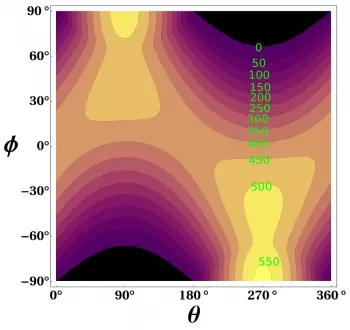Irradiance is the power of solar radiation per unit area. In the international system of units, it is measured in (W/m2 ).

Solar irradiation is the quantity that measures the energy per unit area of ​​incident solar radiation on a surface - the power received during a time (J/m2 or Wh/m2 ).

The term solar radiation is a generic concept, but it is not quantified to any magnitude. The magnitudes that describe the solar radiation that reaches the earth's surface per square meter are irradiance and solar irradiance.

Solar energy is a renewable energy source that depends on the irradiation data parameters to be efficient. Therefore, before investing in a new solar plant, it is necessary to gather the most solar data possible. With this data and the help from a specialist, we will get a solar resource assessment.

The difference is that irradiance measures the power of solar radiation on a surface in an instant. On the other side, irradiation does so over a while. That is, irradiation measures the amount of energy received on a given surface.

## Origin of Solar Irradiance: the Sun.

The origin of radiation that reaches the Earth is the Sun. Solar radiation is the thermal energy released due to the nuclear fusion reaction that occurs inside the Sun.

The energy generated causes the Sun to be a gigantic incandescent mass. The temperature in its layer is about 6000 degrees Celsius.

Being an incandescent body, the Sun emits electromagnetic radiation in a wide range of wavelengths.

There are several types of solar irradiance.

Total solar irradiance measures solar power at all wavelengths per unit area incident in the Earth's upper atmosphere.

It is measured on the Earth's surface at a given location with a surface element perpendicular to the Sun's rays. This type of irradiation excludes diffuse solar radiation.

The direct irradiance is equal to the irradiance outside the Earth's atmosphere minus the atmospheric losses due to absorption and scattering. Losses mainly depend on:

• The time of day (length of the path of light through the atmosphere based on the angle of solar elevation)

• The number of clouds in the sky.

• Humidity.

• Other aspects such as aerosols, ozone molecules, water vapor, etc.

Irradiance on the atmosphere also varies with the time of year. However, this effect is generally less significant compared to the impact of direct radiation losses.

The average direct irradiance from the Sun is 1367 W/m2 at the atmosphere's surface. This magnitude is called the solar constant.

### 3.- Diffuse Horizontal Irradiance or Diffuse Sky

Diffuse horizontal irradiation or diffuse sky radiation is radiation from light scattered by the atmosphere at the Earth's surface. It is measured on a flat surface with radiation from all points in the sky. In this measure, we exclude solar radiation from the solar disk.

If there were no atmosphere, diffuse horizontal irradiation would be practically zero.